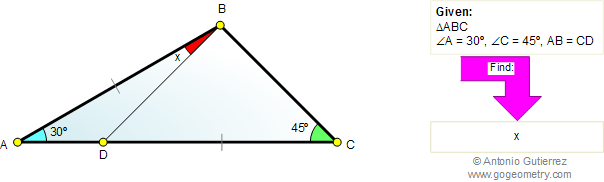# Online Geometry Problem 602: Triangle, Angles, 30 and 45 Degrees, Cevian, Congruence

The figure shows a triangle ABC. The measures of angles A and C are 30 and 45 degrees, respectively. If AB = CD, find the measure of angle ABD.Home | Geometry | Search | Problems | All Problems | 601-610 | Email | Post a comment or solution | By Antonio Gutierrez